## Speed Run!

### DaForce- | Released 2013

Speed Run! (C) DaForce Devs

This game has been abandoned and that means that to give back to the community we are releasing the source code to the game so you can tweak it as you like.

Matthew Allen
Co-Founder , DaForce Devs

Source Code

``````package javagame;

import java.awt.Color;
import java.awt.Image;
import javax.swing.JFrame;
import java.awt.Graphics;
import java.awt.Font;
import java.awt.event.KeyAdapter;
import java.awt.event.KeyEvent;
import java.awt.image.ImageObserver;
import java.io.BufferedWriter;
import javax.swing.ImageIcon;

public class JavaGame extends JFrame implements Runnable{
int x, y, xDirection, yDirection;
private Image dbImage;
private Graphics dbg;
Image face;
boolean customsize;
int resx;
int resy;
boolean dead;
boolean ngame;
int score;
boolean firstkeyup;
boolean firstkeyside;
int speed;
int deaths;
boolean hashit;
boolean counter;
Color myBlackColor;
boolean debug;
String ver;
int date;
int cpu;
int resscore;
int res1;
String os;
Font font = new Font("Arial", Font.BOLD,12);
Font verfont = new Font("Calibri", Font.BOLD,40);
@Override
public void run(){

try{
while(true){
move();
if(dead == false){
if(hashit == true){
deaths = deaths+137;

resscore = resx/100;
resy = resy+100;
}
Thread.sleep(10);
}
}
}
catch(Exception e){
System.out.println("Error");
}
}

public void move(){
x +=xDirection;
y +=yDirection;

if(x <=10){
dead = true;
xDirection = 0;
yDirection = 0;
x = 2000;
y = 2000;

}

if (x >= 1280 - 20){
dead = true;
xDirection = 0;
yDirection = 0;
x = 2000;
y = 2000;
}

if(y <=20){
dead = true;
xDirection = 0;
yDirection = 0;
x = 2000;
y = 2000;
}

if (y >= 720 - 20){
dead = true;
xDirection = 0;
yDirection = 0;
x = 2000;
y = 2000;
}

if(score > 1){
speed = score/12;
}

}

private void xDirection(int i) {
}
public void setXDirection(int xdir){
xDirection = xdir;
}
public void setYDirection(int ydir) {
yDirection = ydir;
}

public class AL extends KeyAdapter {
public void keyPressed(KeyEvent e){
int keyCode = e.getKeyCode();

if(keyCode == e.VK_F){
xDirection = 0;
yDirection = 0;
x = 640;
y = 360;
customsize = false;
dead = false;
ngame = true;
score = 0;
debug = false;
firstkeyup = true;
firstkeyside = true;
hashit = false;
ngame = true;
deaths = 0;
score = 0;
debug = false;
firstkeyup = true;
firstkeyside = true;
counter = false;
hashit = false;
}

if(dead == true){

if(keyCode == e.VK_1){
resx = 1280 ;
resy = 720 ;
customsize = true;

}

if(keyCode == e.VK_2){
resx = 1600;
resy = 900;
customsize = true;
}

if(keyCode == e.VK_3){
resx = 1920;
resy = 1080;
customsize = true;
}
}

if(customsize == true){
setSize(resx, resy);
}

if(keyCode == e.VK_F3){
debug = true;
}

if(keyCode == e.VK_G){
if(dead == true){
System.exit(1);
}
}

if(dead == false){

if(keyCode == e.VK_LEFT){
if(firstkeyup == true){
setXDirection(- +speed);
firstkeyside = true;
firstkeyup = false;
ngame = false;
hashit = true;
score = score+resscore;

}
}
if(keyCode == e.VK_RIGHT){
if(firstkeyup == true){
setXDirection(+ +speed);
firstkeyside = true;
firstkeyup = false;
ngame = false;
hashit = true;
score = score+resscore;
}
}

if(keyCode == e.VK_UP){
if(firstkeyside == true){
setYDirection(- +speed);
firstkeyside = false;
firstkeyup = true;
ngame = false;
hashit = true;
score = score+resscore;
}
}

if(keyCode == e.VK_DOWN){
if(firstkeyside == true){
setYDirection(+ +speed);
firstkeyside = false;
firstkeyup = true;
ngame = false;
hashit = true;
score = score+resscore;
}
}
}
}
}

public JavaGame(){
//Load images
ImageIcon i = new
ImageIcon("/face.png");
face = i.getImage();
face = i.getImage();
//game props
resx = 1280 ;
resy = 720 ;

ver = "Alpha 1.2.5";
addKeyListener(new AL());
setTitle("Speed Run! - " +ver);
setSize( resx, resy);
setResizable(false);
setVisible(true);
setDefaultCloseOperation(JFrame.EXIT_ON_CLOSE);
setAlwaysOnTop(true);
setLocationRelativeTo(null);
customsize = false;
dead = false;
ngame = true;
score = 0;
debug = false;
firstkeyup = true;
firstkeyside = true;
hashit = false;
x = resx/2;
y = resy/2;

}

public void paint(Graphics g){
dbImage = createImage(getWidth(), getHeight());
dbg = dbImage.getGraphics();
paintComponent(dbg);
g.drawImage(dbImage,0, 0, (ImageObserver) this);
}

public void paintComponent(Graphics g){
g.setColor(Color.GRAY);
g.fillRect(0, 20, resx, 28);  // HOR AXIS , VERT AXIS , WIDTH
g.drawImage(face, x+11, y+11, (ImageObserver) this);
g.setColor(Color.black);
g.fillRect(0, 20, resx, 25);  // HOR AXIS , VERT AXIS , WIDTH

g.setColor(Color.white);
g.setFont(verfont);
g.drawString(""+ver,20 , resy-20);
g.setFont(font);
//g.drawString("Speed : "+speed, 1130, 40);
g.drawString("Score : "+deaths,600 , 40);

if(dead == true){
g.setFont(font);
g.drawString("DEAD", 10, 40);
g.drawString("PRESS 'F' to RESTART ", 170, 40);
g.drawString(" PRESS 'G' to EXIT", 330, 40);
setBackground(Color.RED);
x = resx/2;
y = resy/2;
xDirection = 0;
yDirection = 0;
}

//if (dead == false){
//g.drawString("LIVING", 10, 40);
//g.drawString("Coord:("+x+","+y+") |", 50, 40);
//}

if(debug == true){
setFont(font);
g.setColor(Color.DARK_GRAY);
g.fillRect(15, 70, 150, 230);  // HOR AXIS , VERT AXIS , WIDTH
g.setColor(Color.WHITE);
g.drawString("DEBUG MONITOR", 20, 85);
g.drawString("X : "+x, 20, 100);
g.drawString("Y : "+y, 20, 115);
g.drawString("-----------------------", 20, 125);
g.drawString("Dead : "+dead, 20, 135);
g.drawString("NGame : "+ngame, 20, 150);
g.drawString("Score : "+deaths, 20, 165);
g.drawString("Speed : "+speed, 20, 180);
g.drawString("Hashit (Has Hit) : "+hashit, 20, 195);
g.drawString("Firstkeyup : "+firstkeyup, 20, 210);
g.drawString("Firstkeyside : "+firstkeyside, 20, 225);
g.drawString("Version : "+ver, 20, 240);
g.drawString("No. of CPUs:"+cpu, 20, 270);
g.drawString("OS : "+os, 20, 285);

os = System.getProperty("os.name");
cpu = Runtime.getRuntime().availableProcessors();
}

if(ngame == true){
dead = false;
g.setFont(font);
g.drawString("Welcome to 'Speed Run!'", 300, 200);
g.drawString("Touching the outer edges of the screen will result in your death ,", 300, 225);
g.drawString("Use the arrow keys to control your character, F to restart and G to Quit.", 300, 250);
g.drawString("The catch? You cannot press an arrow key that is opposite the last key you pressed. For example, Up - Down will not work , Up - Left will", 300, 275);
g.drawString("Good Luck, Press an arrow key to begin", 300, 300);
score = 1;
speed = 1;
setBackground(Color.orange);
setYDirection(0);
setXDirection(0);
x = 640;
y = 360;
}
repaint();
}

public static void main(String[] args){
System.out.println("JavaGame main succesulfully launched");
JavaGame jg = new JavaGame();
Thread t1 = new Thread(jg);
t1.start();
}
}

``````

Articles

#### Speed Run! 1.2.5 - New Resolutions and Debugging tools

News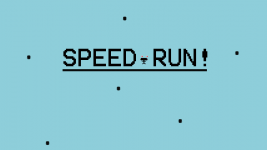Hello everyone.
Alpha 1.2.5 has been released for Speed Run!Why 1.2.5 not 1.3? as announced in 1.5 version 1.3 would implement either enemy's or another form of object to add difficulty to the game and that is still coming.
So what did we change in 1.2.5?Support for 3 preset resolutions : 720P , 900P , 1080P all at a 16:9 aspect ratio.
Removed many untidy and unused pieces of code that was using up resources.Fixed various glitches with respawning.
Added debug menu (Activated by F3)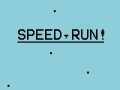#### Speed Run! ALPHA 1.2

News

Speed Run! is a 2D Game developed in java. It is currently in alpha with many features to be added. The games basic form is currently development in the...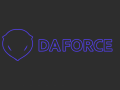#### Alpha 1.1 Release

News

Speed Run! (C) Da Force Devs. Speed Run! is our first release and is currently in alpha. The main game mechanic is the concept of staying within the screen...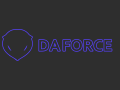#### Alpha Release

News

Speed Run! is now being released for download as an alpha package. At this point in time the game is a free download and being distributed under the freeBSD...

#### Post article >>

Files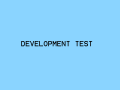#### Speed Run! ALPHA 1.2.5 - UNSTABLE

Full Version

Hello everyone. Alpha 1.2.5 has been released for Speed Run! Why 1.2.5 not 1.3? as announced in 1.5 version 1.3 would implement either enemy's or another...#### Speed Run! ALPHA 1.2

Full Version 3 comments

Speed Run! is a 2D Game developed in java. It is currently in alpha with many features to be added. The games basic form is currently development in the...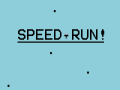#### Speed Run! ALPHA 1.1

Full Version

Download for Speed Run! ALPHA 1.1 CHANGES: Cleaned up code Increased performance (wasn't an issue) Added more GUI Added Living/Dead text at top left Added...#### Speed Run! ALPHA

Demo

Alpha download of Speed Run! This is the very first release of the game and thus is very lacking in gameplay.As it is many features will be added.

#### Add file >>

Post a comment
Sign in or join with:

Only registered members can share their thoughts. So come on! Join the community today (totally free - or sign in with your social account on the right) and join in the conversation.

Profile
##### Icon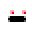##### Share
Community Rating

Average

-

0 votes submitted.

You Say

-

Ratings closed.

Style
##### Project
Twitter

Latest tweets from @daforcedevs

SpeedRun - Java: Youtu.be via @YouTube

Jul 6 2013

Speed Run! 1.2 is now out Indiedb.com

Apr 30 2013

First release of speedrun now up!http://www.indiedb.com/members/daforcedevs

Apr 28 2013

DaForce Devs is now on twitter, we are an aspiring indie studio.

Apr 26 2013

Tags
Embed Buttons
Link to Speed Run! by selecting a button and using the embed code provided more...
Statistics
##### Articles
You may also like